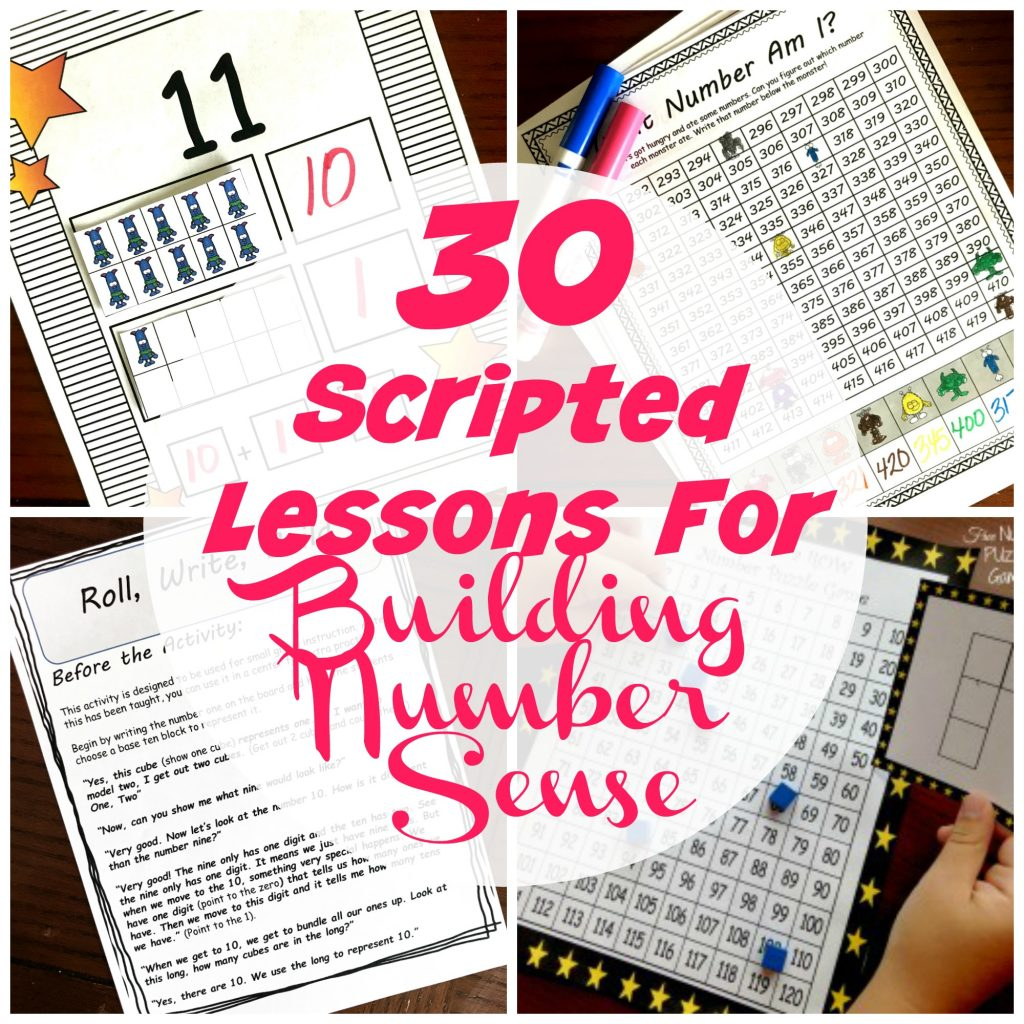# Why You Should Use Math Manipulatives With Upper Grade Homeschoolers

We love using math manipulatives with our younger math students, but we tend to shy away from using them with our older ones. Check out why they should be used!

Children counting teddy bears.

Little ones filling ten frames up with themed erasers.

Hundreds charts are used and counted on daily.If you walk into a K – 2 classroom or purchase a homeschool curriculum for this age, you expect to see each of the following. We instinctively know that children need to manipulate and explore to understand math. They need to see what 10 looks like in ten frames, on a hundreds chart, and using base ten blocks. They need to manipulate items to understand what happens when we add versus subtract.

You see, no one has a problem with this type of exploration taking place in the younger grades.

We let the children “play” and naturally know that this is developmentally appropriate and that they will not need this type of exploration forever. At some point, they grasp what adding and subtracting is and they can do it without fingers or manipulatives. Before long, they develop number sense and understand the difference of 23 and 32 and no longer need to show them with base ten blocks.

We use the manipultives to help build number sense in our children and know that they will not always need these tools to solve problems.

If you are looking for a way to incorporate Number lines, base ten blocks, hundreds charts, and more into your math time…..check out these 30 scripted lessons that will help you build number sense in you k – 2 students.## Using Math Manipulatives In Upper Grades

But something happens when our children get older and start exploring fractions, decimals, and harder multiplication and division

We just want to teach a formula.

We only want to provide them the steps to solve the problems.

And we definitely don’t want to stray from the traditional method of teaching math.

BUT is this what is best for our kiddos?

#### Building a Foundation

Just as we use manipulatives to build number sense in our little ones, we can still use manipulatives with our older ones too. And it is just for building that foundation.

When I first introduce multiplying fractions, I have a choice. I can just teach the formula, or I can require my oldest to model multiplying fractions

I want my kiddo to understand what is happening when I multiply two fractions together. So I need him to know WHY he is getting a smaller answer when fractions are multiplied, and this is best done through modeling with arrays.

There is a time and place to teach the formula and to practice that formula. But there is also a time and place to teach the WHY behind the math. To develop that fraction sense, decimal sense, or number sense. To build a strong foundation.

And once that foundation is established, we can drop modeling or the “new math” and just solve the problem. Just as a second-grader no longer needs a ten frame to solve a subtraction problem, our older children will no longer need a model to help solve the problem.

And they might surprise you and be able to do it in their head.

Why?

Because you have developed a strong math foundation!

### Visual Learners

Did you know that over 65% of the population are visual learners?

This means that many children can not be presented with a formula and understand the math behind it.

It wasn’t until I sat in a class on how to teach math (age 20) did I understand how subtraction with regrouping worked. When the base ten blocks came out, and for the first time I visually saw what was happening, a light bulb came on.  I FINALLY understood the formula.

This clear visual was the beginning of a much deeper understanding of math. And when I could visual the numbers in my head, I could for the first time in my life do mental math.

Providing a visual of the math they are doing does not replace the formula. It is just a stepping stone to help our children “see” what is happening when they work through the formula.

## Steps To Take:

If you are worried that your child is not understanding math, I want to encourage you to try doing a little more modeling….even if they are older.

Take it from a person who thought she was bad at math. From a young lady that couldn’t do simple math without fingers, a piece of paper, or a calculator.

When I began to see math modeled, understanding began to set in. Now I blog about math and can actually do some mental math.

But you may ask, where can I find ways to model harder math? Here are a few.

1. First, check out YouTube videos. There are so many that will model the math concept you are trying to teach your student.
2. Look for activities that allow your children to practice modeling math. You’ve Got This Math and Math Geek Mama has numerous freebies that will let your children get in some fun practice with modeling.
3. Or you could even try different math curriculums where the focus is on modeling and then mastering formulas. Math Mammoth is a very inexpensive one that does a great job of modeling math and using math manipulatives.

It may take you out of your comfort zone, but this step is so important. Using math manipulatives with your older children is totally worth it and so beneficial.

You’ve Got This!